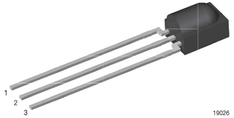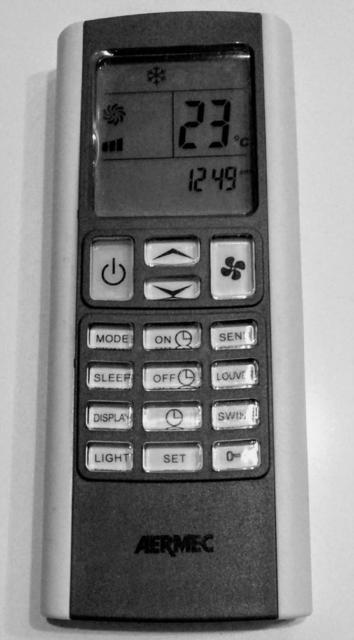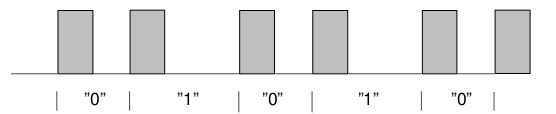# AERMEC A/C remote controls: IR protocol reverse engineering

Why would you ever want to know the IR protocol between an air conditioner and its remote control?

If you do this, it would then be easy to turn your A/C into an IP-controlled device!

If you know how to craft the signal, you could send it using a different controller: for example, an ESP8266 with MQTT and an IR led.

The first step is being able to read the signal in some form, then figure out how the bits are represented, and finally understand the structure of the messages (i.e. how operating mode, temperature, fan speed, etc. are encoded into the bit sequence).

Hardware setup:

• IR receiver module (a 3 pin component, I used a HS38B3)
• Arduino UNO

Software setup:

• Arduino IDE
• IRremote library1 = OUT, 2 = GND, 3 = Vs

The connections are quite straightforward: pin 1 to an Arduino digital pin (e.g. 11), pin 2 to Arduino GND, pin 3 to Arduino 5V.

Install the IRremote library, then copy the IRrecvDumpV2 example in Arduino IDE, compile and flash. Start the serial monitor, grab your remote control and see if something happens when you press remote keys.

IRrecvDumpV2 tries to guess if your remote is among the known ones, and dumps the raw timings (marks and spaces) of the entire signal.

An A/C remote control is usually different from e.g. a TV remote: while the latter just encodes the key, and acts like a keyboard, the former encodes all the operating parameters and sends them all in every message. For example, when you set a new target temperature, the remote not only sends the temperature, but also the operating mode, fan speed, scheduled start and stop time, etc.

### Aermec RC model C123During the initial runs of IRrecvDumpV2, the first thing that needed attention was that the array of raw timings was overflowing: the signal was too long for the array default size (101).

The relevant configuraton is in `IRremoteInt.h`, where RAWBUF is defined: `#define RAWBUF  255`

With this new setting, I was finally able to get the timings of the whole signal. After spending some time trying to figure out recurring patterns in the signal, a few facts emerged:

• the base duration of both marks and spaces is 950 usec; all other durations are a multiple of this base duration
• the signal always starts with a mark of 3x base durations, followed by a space of 3x base durations
• the signal ends with a mark of 3x base durations
• in the middle of the signal, the pattern 3x mark 3x space occurs twice: this pattern is used as a separator, so that the overall signal structure is: [start pattern] + message1 + [separator] + message2 + [separator] + message3 + [end mark]
• message1 is equal to message2 and message3 (i.e. the message is repeated three times)
• within the message, no mark exceeds 2x base durations, and no space exceeds 2x base durations

The last observation indicates that bits are probably encoded using bi-phase coding. Every bit takes 2x base durations. "0" is mark+space (falling edge), "1" is space+mark (rising edge).When "1" is followed by a "0", you get a 2x base duration mark. When "0" is followed by a "1", you get a 2x base duration space.

Every message is 80x base durations long. With bi-phase coding, this means encoding 40 bits, i.e. 5 bytes.

Unfortunately, none of the examples/protocols in IRremote library seemed to decode a bi-phase encoded signal, so I wrote my own decode function, following the useful guidance in `ir_Template.cpp`:

`#define BITS          40  // The number of bits in the command  #define HDR_MARK    2850  // The length of the Header:Mark #define HDR_SPACE1  3800  // The lenght of the Header:Space when first data bit = 1 #define HDR_SPACE0  2850  // The lenght of the Header:Space when first data bit = 0  #define HALF_BIT_US  950  // The length of a half bit // match_mark and match_space rewritten to use a fixed tolerance, // not a percentage of mark/space duration#define USEC_TOLERANCE  250 // Due to sensor lag, when received, Marks tend to be 100us too long int  match_mark (int measured_ticks,  int desired_us) {   bool passed = (((measured_ticks*USECPERTICK - MARK_EXCESS) >= (desired_us - USEC_TOLERANCE))                 && ((measured_ticks*USECPERTICK - MARK_EXCESS) <= (desired_us + USEC_TOLERANCE)));   return passed; }  // Due to sensor lag, when received, Spaces tend to be 100us too short int  match_space (int measured_ticks,  int desired_us) {   bool passed = (((measured_ticks*USECPERTICK + MARK_EXCESS) >= (desired_us - USEC_TOLERANCE))                 && ((measured_ticks*USECPERTICK + MARK_EXCESS) <= (desired_us + USEC_TOLERANCE)));   return passed; }  #if DECODE_AERMEC bool  IRrecv::decodeAermec (decode_results *results) {     // unsigned long  data   = 0;  // Somewhere to build our code     unsigned short hbit;     byte data;     int offset = 1;  // Skip the Gap reading     int hbits = 0;      // Check we have the right amount of data     // if (irparams.rawlen != 1 + 2 + (2 * BITS) + 1)  return false ;      // Check initial Mark+Space match     if (!match_mark(results->rawbuf[offset++], HDR_MARK ))  return false ;      if (match_space(results->rawbuf[offset], HDR_SPACE1)) {         hbits = 1;         hbit = 0; // 1st half bit = 0     }     else if (!match_space(results->rawbuf[offset], HDR_SPACE0)) return false;     offset++;      // Read the half bits in     while (hbits < 255) {         if (match_mark(results->rawbuf[offset], HALF_BIT_US)) {             // data = (data << 1) | 1 ; // push 1             hbit[hbits] = 1;             hbits++;         }         else if (match_mark(results->rawbuf[offset], HALF_BIT_US*2)) {             // data = (data << 2) | 3 ; // push 11             hbit[hbits] = 1; hbit[hbits+1] = 1;             hbits+=2;         }         else if (match_mark(results->rawbuf[offset], HALF_BIT_US*3)) {             // data = (data << 3) | 7 ; // push 111             hbit[hbits] = 1; hbit[hbits+1] = 1; hbit[hbits+2] = 1;             hbits+=3;         }         else if (match_mark(results->rawbuf[offset], HALF_BIT_US*4)) {             // data = (data << 4) | 15 ; // push 1111             hbit[hbits] = 1; hbit[hbits+1] = 1; hbit[hbits+2] = 1; hbit[hbits+3] = 1;             hbits+=4;         }         else return false;         offset++;          if (hbits < 255) {           if (match_space(results->rawbuf[offset], HALF_BIT_US)) {             // data = (data << 1) | 0 ; // push 0             hbit[hbits] = 0;             hbits++;           }           else if (match_space(results->rawbuf[offset], HALF_BIT_US*2)) {             // data = (data << 2) | 0 ; // push 00             hbit[hbits] = 0; hbit[hbits+1] = 0;             hbits+=2;           }           else if (match_space(results->rawbuf[offset], HALF_BIT_US*3)) {             // data = (data << 3) | 0 ; // push 000             hbit[hbits] = 0; hbit[hbits+1] = 0; hbit[hbits+2] = 0;             hbits+=3;           }           else if (match_space(results->rawbuf[offset], HALF_BIT_US*4)) {             // data = (data << 4) | 0 ; // push 0000             hbit[hbits] = 0; hbit[hbits+1] = 0; hbit[hbits+2] = 0; hbit[hbits+3] = 0;             hbits+=4;           }           else return false;           offset++;         }     }     DBG_PRINTLN("Half-bits sequence:");     for (int i = 0; i<255; i++) DBG_PRINT(hbit[i]);     DBG_PRINTLN("");      // check for separator mark and space (3 half-bits mark, 3 half-bits space)     if (!hbit || !hbit || !hbit) return false;     if (hbit || hbit || hbit) return false;      if (!hbit || !hbit || !hbit) return false;     if (hbit || hbit || hbit) return false;         DBG_PRINTLN("111000 mid sep passed.");     // check for end mark (3 half-bits)     if (!hbit || !hbit || !hbit) {         DBG_PRINTLN("111 end mark failed.");         return false;     }      int koff[] = {0, 86, 172};      for (int k = 0; k<3; k++) {       for (int b = 0; b<5; b++) {          for (int i = 0; i<8; i++) {             offset = koff[k] + ((b*8+i)*2);             if ( !(hbit[offset]) && (hbit[offset+1]) )      // 0->1 transition is bit 1                 data[k*5+b] = (data[k*5+b] << 1) | 1 ;             else if ( (hbit[offset]) && !(hbit[offset+1]) ) // 1->0 transition is bit 0                 data[k*5+b] = (data[k*5+b] << 1) | 0 ;             else return false;                              // anything else aborts decoding         }       }     }     DBG_PRINTLN("Bit seq:");     for (int i = 0; i<15; i++) {         DBG_PRINT(data[i], HEX);         DBG_PRINT(" ");     }     DBG_PRINTLN("");          for (int b = 0; b<5; b++) {         if ( (data[b] != data[b+5]) || (data[b] != data[b+10]) ) return false;     }     unsigned long val = 0;     val += data;    val = val << 8;     val += data; val = val << 8;     val += data; val = val << 8;     val += data;     // Success     results->bits        = BITS;     results->address     = data;     results->value       = val;     results->decode_type = AERMEC;     return true; } #endif`

The decodeAermec function is far from being optimized and is not a beautiful piece of code, but it works.

To overcome the fact that the decoded value is a 32 bit integer (and 40 were needed), I put the first byte in the "address" property of the results structure.

With the correct decode function in place, it was time to play with the remote control, looking at how bits changed when operating on a given setting.

For example, the message for 24°C, fan auto, mode cool, no power-on timer and no power-off timer is 1C:12:00:00:06.

If you set "sleep" mode in addition, the message becomes: 1C:13:00:00:06.

If you take the temperature setting to 23°C (sleep mode still on): 1C:11:00:00:06.

Removing "sleep" mode, at 23°C, the reading becomes: 1C:10:00:00:06.

#### First nibble (bit 0 to 3): power on/off and operating mode

 1xxx power on/off 0001 mode: cool 0010 mode: heat 0101 mode: fan 0100 mode: dry (dehumidifier) 0011 mode: auto [triangle of arrows] 0000 off and timer set (?)

#### Second nibble (bit 4 to 7): fan speed and "I feel"

 00xx fan speed 1 (low) 01xx fan speed 2 (med) 10xx fan speed 3 (high) 11xx fan speed AUTO xx1x "I feel" mode set xxx0 (not used, always 0)

#### 2nd byte: temperature and "sleep" mode

 hex bin use / description 00 0000 000x 15°C set temp 20 0010 000x 16°C set temp 40 0100 000x 17°C set temp 60 0110 000x 18°C set temp 80 1000 000x 19°C set temp A0 1010 000x 20°C set temp C0 1100 000x 21°C set temp E0 1110 000x 22°C set temp 10 0001 000x 23°C set temp 12 0001 001x 24°C set temp 14 0001 010x 25°C set temp 16 0001 011x 26°C set temp 18 0001 100x 27°C set temp 1A 0001 101x 28°C set temp 1C 0001 110x 29°C set temp 1E 0001 111x 30°C set temp xxxx xxx1 "sleep" mode set

#### 3rd byte: power-on timer

Central unit has no clock: timers are countdowns starting from "now", measured in 10 minutes steps.
10' ahead is 0x01, 50' ahead is 0x05, but 1h ahead is 0x08 (a +3 increment instead of +1: this happens for every hour).

See 3rd byte.

#### 5th byte: fan "strong" bit

Last nibble seems to carry no information, and is always 0110 (6).

 hex bin use / description 06 0000 0110 normal 16 0001 0110 fan "strong" bit set

### Aermec RC model A01

Hoping that all Aermec remote controls would behave the same, I tested another RC, only to find out that it is completely different: even bit encoding is not the same!Looking at the mark and space timings, where marks all have the same duration and spaces two possible durations, this is very likely a "pulse distance modulation".Currently unrated

Comment awaiting approval 3 years, 8 months ago

### New Comment

required

required (not published)

optional

required